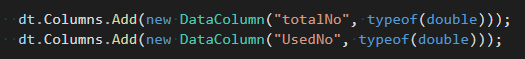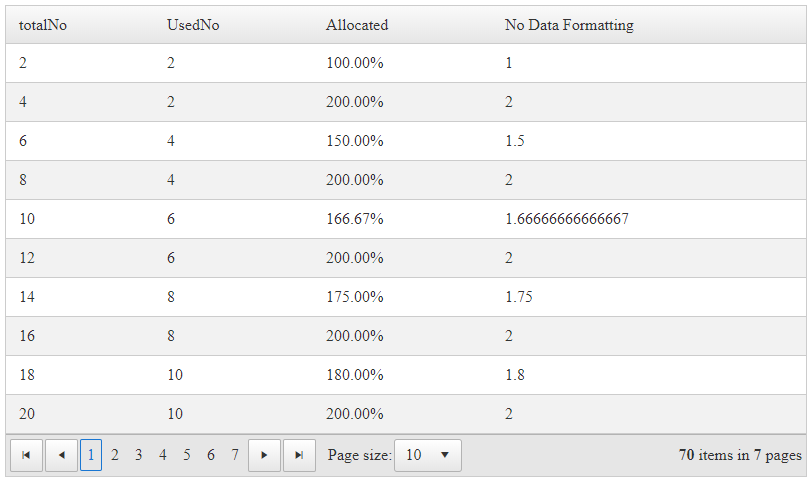# Casting in GridCalculatedColumn - Possible?

1. ##### Marcus8 posts
Member since:
Aug 2019

Posted 12 Jun 2020 Link to this post

Hello All,

I'm trying to calculate a percentage in a GridCalculatedColumn, but both input types are integer, so the return value is incorrect (an int formatted as %, which results in 0%, 100% 200% etc.)

Is it possible to cast the value in the expression, or do I have to do it the hard way using itemdatabound or similar?

markup:
Expression="({0} / {1})" ColumnGroupName="Compute" DataFormatString="{0:P}" />

I tried "(Convert.ToDecimal({0}) .... but that gave me errors.

M.

2. ##### Attila Antal591 posts

Posted 17 Jun 2020 Link to this post

Hi Marcus,

The Calculated column will take into account the type of data of the fields. For instance, the fields bound to RadGrid will need to be of the correct type if you're looking to work with decimals.Then you can use an expression to calculate the numbers you need.

```<telerik:GridCalculatedColumn DataFields="totalNo,UsedNo" HeaderText="Allocated"  Expression="({0} / {1})" ColumnGroupName="Compute" DataFormatString="{0:P}" />
<telerik:GridCalculatedColumn DataFields="totalNo,UsedNo" HeaderText="No Data Formatting"  Expression="({0} / {1})" ColumnGroupName="Compute"  />```

ResultYou can also format that in the code behind and change the cell's values programmatically, see Accessing Cells.

Kind regards,
Attila Antal
Progress Telerik